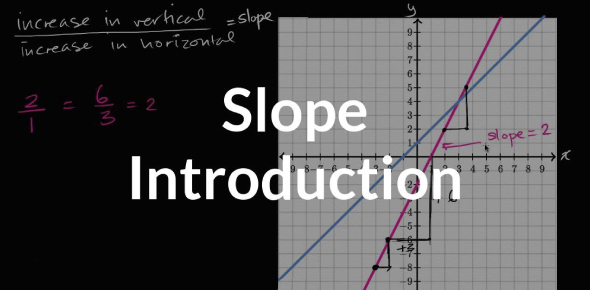# Math Exam On Slope! Trivia Quiz

20 Questions | Attempts: 397
ShareSettings• 1.
Matthew wants to rent a limousine for the prom.  He calls the company to find out that the cost is \$150 plus \$0.25 per mile.  Which equation below shows the cost, y, of renting the limousine for x miles?
• A.

Y = (150 + .25)x

• B.

Y = 15x + .25

• C.

Y = 150 - .25x

• D.

Y = .25x + 150

• 2.
Which equation below will have a graph that is parallel to the graph of y = -2x + 6
• A.

Y = -2x - 6

• B.

Y = 2x - 6

• C.

Y = 6x - 2

• D.

Y = -6x + 2

• 3.
For the function f(x) = 2x + 5, find the range when the domain is { 0, 1, 2, 3}.
• A.

{0, 1, 2, 3}

• B.

{7, 9, 11, 13}

• C.

{5, 7, 9, 11}

• D.

None of theses

• 4.
Which of the following equations represents a horizontal line?
• A.

X = 7

• B.

Y = 7

• C.

Y = 3x - 4

• D.

2x + y = 5

• 5.
Which of the following sets of ordered pairs is NOT a function?
• A.

(4, 3) (5, 4) (6, 5) (-4, 6)

• B.

(3, 7) (4, 6) (3, -7) (6, 0)

• C.

(2, 3) (4, 3) (5, 5) (6, 5)

• D.

(0, 5) (5, 0) (1, 2) (2, 3)

• 6.
• 7.
Given the graph below, which inequality matches the graph?
• A.

Y

• B.

Y≥-x+1

• C.

Y≤x+1

• D.

Y≤-x+1

• 8.
• A.

Y = 1/2x - 5

• B.

Y = 2x - 4

• C.

Y = -1/2x - 5

• D.

Y = x + 1

• 9.
Which equation below contains the ordered pairs (1, 2) and (4, 5)?
• A.

Y = -x + 9

• B.

Y = -x - 9

• C.

Y = x + 9

• D.

Y = x + 1

• 10.
A softball league charges each team \$350 plus \$10 per player. Which table below shows the correct relationship        between the number of players and the team cost?
• A.

Option 1

• B.

Option 2

• C.

Option 3

• D.

Option 4

• 11.
A mobile telephone company charges a flat monthly rate of \$25 per month plus \$0.25 per minute of airtime.  How many minutes did Linda use her cellular phone if her telephone bill was \$56?
• A.

112 Minutes

• B.

124 minutes

• C.

156 minutes

• D.

200 minutes

• 12.
Jake started with \$75 in his savings account.  Each week he withdraws \$10 to use as spending money.  Which equation best describes a, the amount in Jake’s savings account after a certain number of weeks, w?
• A.

A = 75w - 10

• B.

A = 75w + 10

• C.

A = 75 - 10w

• D.

A = 10w - 75

• 13.
Which of the equations below represents the second step of the solution process?
• A.

5(5x + 7) + 3 = – 78

• B.

5(5x + 10) = – 78

• C.

25x + 15 + 7 = – 78

• D.

25x + 3 + 7 = – 78

• 14.
Solve for y: 4x + 2y = 6
• A.

Y = -2x + 3

• B.

Y = 2x + 3

• C.

Y = 2x + 6

• D.

Y = -2x + 6

• 15.
Which equation below best describes the graph below?
• A.

3x - y = 6

• B.

2x + y = -6

• C.

2x - 3y = -12

• D.

-2x + 3y = 4

• 16.
Megan decided to earn some extra money for  the holidays by wrapping gifts at the mall.  She would earn \$15 plus \$0.50 for each package she wrapped.  The equation for this situation is                                  y = 0.50x + 15 What is the rate of change and what does it  represent in this problem?
• A.

\$0.50.the amount earned for each package

• B.

\$0.50, amount earned for coming to work

• C.

\$15, amount earned for each package

• D.

\$15, amount earned for coming to work

• 17.
Solve the equation for y: 3x - 3y = 12
• A.

Y = -x + 4

• B.

Y = -x - 4

• C.

Y = x - 4

• D.

Y = x + 12

• 18.
Which of the following represents a function?
• A.
• B.
• C.
• D.

X = 6

• 19.
If (c, 5c) amd (3c, 2c) are two points on the graph of a line, and c is not equal to 0, what is the slope of the line?
• 20.
Which graph does not represent y as a function of x?
• A.
• B.
• C.
• D.

## Related TopicsBack to top
×

Wait!
Here's an interesting quiz for you.